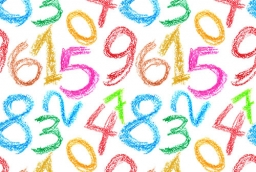# Evaluate expression

Calculate the value of the expression z/3 - 2 z/9 + 1/6, for z = 2

x =  0.3889

### Step-by-step explanation:Did you find an error or inaccuracy? Feel free to write us. Thank you!Tips to related online calculators
Need help to calculate sum, simplify or multiply fractions? Try our fraction calculator.

## Related math problems and questions:

• Expression plus minusEvaluate expression: (-1)2 . 12 – 6 : 3 + (-3) . (-2) + 22 – (-3) . 2
• Evaluate mixed expressionsWhich of the following is equal to 4 and 2 over 3 divided by 3 and 1 over 2? A. 4 and 2 over 3 times 3 and 2 over 1 B. 14 over 3 times 2 over 7 C. 14 over 3 times 7 over 2 D. 42 over 3 times 2 over 31
• Evaluate expressionEvaluate expression using BODMAS rule: 1 1/4+1 1/5÷3/5-5/8
• Evaluate expression 2Evaluate expression with negatives: (-3)+4+(-8)+(-6)+4+(-1)
• Mixed numbersRewrite mixed numbers, so the fractions have the same denominator: 5 1/5 - 2 2/3
• Fractions and mixed numerals(a) Convert the following mixed numbers to improper fractions. i. 3 5/8 ii. 7 7/6 (b) Convert the following improper fraction to mixed number. i. 13/4 ii. 78/5 (c) Simplify these fractions to their lowest terms. i. 36/42 ii. 27/45 2. evaluate following ex
• Simplify 3Simplify mixed numerals expression: 8 1/4- 3 2/5 - (2 1/3 - 1/4) Show your solution.
• Evaluate expr with 2 varsIf a=6 and x=2, find the value of (2ax + 7x- 10) /(4ax-3a-2)
• Evaluate 11Multiply the quotient of 6 and 2 by 3, then add 1. Add 1 and 6, then divide by 2 and multiply by 3. Divide 6 by the product of 2 and 3, then add 1.
• Equivalent fractions 2Write the equivalent multiplication expression. 2 1/6÷3/4
• Simplify 7Simplify. 7-1/3÷ 3-2/3 of 2+ 4-1/2÷ 2-1/4+ 1/2 solution and by step by step
• FractionsSort fractions z1 = (6)/(11); z2 = (10)/(21); z3 = (19)/(22) by its size. Result write as three serial numbers 1,2,3.
• EvaluateThe division of numbers $a and$b increase by-product of the numbers $c and$d
• Evaluate 17Evaluate 2x+6y when x=- 4/5 and y=1/3. Write your answer as a fraction or mixed number in simplest form.
• RunningJoanne ran 8 1/2 Kyleigh 1 2/3 and John 6 3/4 miles in one day. How many miles did the girls run in 3 days?
• Fractions 3Calculate 1/9 of 27:Add two mixed fractions: 2 4/6 + 1 3/6